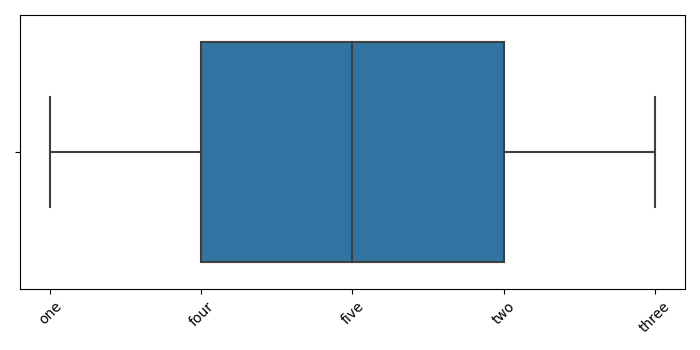# Rotate xtick labels in Seaborn boxplot using Matplotlib

To rotate xtick labels in Seaborn boxplot, we can take the following steps −

• Create data points for xticks.

• Draw a boxplot using boxplot() method that returns the axis.

• Now, set the xticks using set_xticks() method, pass xticks.

• Set xticklabels and pass a list of labels and rotate them by passing rotation=45, using set_xticklabels() method.

• To display the figure, use show() method.

## Example

import seaborn as sns
from matplotlib import pyplot as plt
plt.rcParams["figure.figsize"] = [7.00, 3.50]
plt.rcParams["figure.autolayout"] = True
xticks = [1, 4, 5, 2, 3]
ax = sns.boxplot(xticks)
ax.set_xticks(xticks)
ax.set_xticklabels(["one", "two", "three", "four", "five"], rotation=45)
plt.show()

## Output